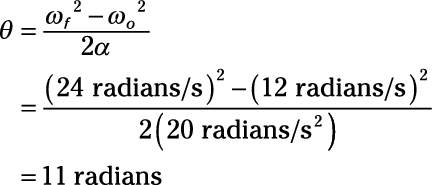##### Physics I Workbook For Dummies with Online Practice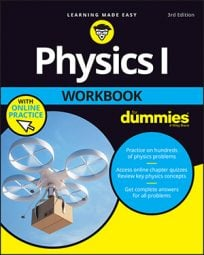You can connect angular displacement, angular velocity, and angular acceleration. The corresponding equation for linear motion is vf2vo2 = 2as. Substituting omega for v, alpha for a, and theta for s gives you: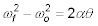Use this equation when you want to relate angle to angular velocity and angular acceleration.

## Sample question

1. A merry-go-round slows down from 6.5 radians/s to 2.5 radians/s, undergoing an angular acceleration of 1.0 radians/s2. How many radians does the merry-go-round go through while this is happening?2. Solve for theta: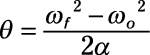3. Plug in the numbers: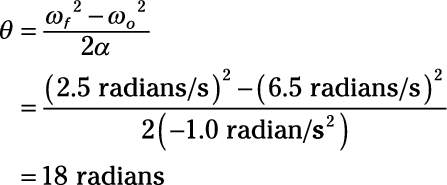## Practice questions

If the angular acceleration is 10 radians/s2, what is the total angle the blades have gone through?

2. Your ball on a string is traveling around in a circle.

If it goes from 12 radians/s to 24 radians/s and the angular acceleration is 20 radians/s2, what is the total angle the ball has gone through during this acceleration?

Following are answers to the practice questions:

1. Use this equation:2. Solve for theta:3. Plug in the numbers: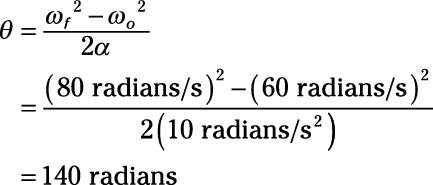1. Use this equation:2. Solve for theta:3. Plug in the numbers: